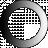# American Dictionary of the English Language

Dictionary Search

### Exponent

EXPO'NENT, noun [Latin exponens; expono, to expose or set forth; ex and pono, to place.]

1. In algebra, the number or figure which, placed above a root at the right hand, denotes how often that root is repeated, or how many multiplications are necessary to produce the power. Thus a denotes the second power of the root a, or aa: a denotes the fourth power. The figure is the exponent or index of the power.

2. The exponent of the ratio or proportion between two numbers or quantities, is the quotient arising when the antecedent is divided by the consequent. Thus six is the exponent of the ratio of thirty to five.